# MIRACLE data analysis tools

## 5)Combined analysis of ground magnetic and ionospheric electric field data using the method of characteristics ("JEQ-based" method of characteristics):

### - Short description of the analysis method:

The "JEQ-based" method of characteristics in an spatial inversion method of ground-based data of the magnetic field disturbance and the ionospheric electric field, to obtain distributions of ionospheric conductances and currents. In contrast to the previously used "trial and error" modeling (i.e., guesses of the desired ionospheric quantities are gradually improved until the measured quantities calculated from these models fit the real measurements to a given degree of error), this method is a forward one, i.e., the desired quantities are calculated by solving a differential equation whose coefficients are determined by the measurements. The primary output of the method is the Hall conductance, from which together with the inputs, the ionospheric currents and field-aligned currents (FACs) can be calculated. While other methods ("trial and error", KRM, AMIE) need guesses or statistical inputs for both Hall and Pedersen conductances separately, the method of charactistics only needs an estimate of the Hall to Pedersen conductance ratio. This can be assessed from the level of ground magnetic disturbance, supported by all-sky camera or satellite data if available.

For more details: see Literature (below)

### - Input data requirements:

Since the method provides spatial output distributions, also spatial input data of the ground magnetic and ionospheric electric field is required. This means:
• The method can only be performed in the area where both input data sets exist, i.e., northern Fennoscandia (see MIRACLE map).
• Good backscatter from both STARE radars must be available to obtain electric field vectors for all or most of the common field of view of the radar; this is of particular importance in the southern part of the STARE common field of view, where the magnetometers are located (most restrictive requirement)
• All IMAGE stations must be available (typically 3-6 months after the event).
Furthermore, for small analysis areas like it is the case here, the uniqueness of the results will depend on the electric field structure (coarse rule: the more divergences of E are in the analysis area, the better).

### - Output quantities:

Spatial distributions of ionospheric conductances, currents and field-aligned currents.

### - Literature:

• Amm, O., Direct determination of the local ionospheric Hall conductance distribution from two- dimensional electric and magnetic field data: Application of the method using models of typical ionospheric electrodynamic situations, J. Geophys. Res., 100, 24173, 1995.
• Amm, O., Method of characteristics in spherical geometry applied to a Harang discontinuity situation, Ann. Geophys., 16, 413, 1998.
• Amm, O., P. Janhunen, H.J. Opgenoorth, T.I. Pulkkinen, and A. Viljanen, Ionospheric shear flow situations observed by the MIRACLE network, and the concept of Harang Discontinuity, submitted to AGU monograph on Magnetospheric Current Systems, Geophysical Monograph 118, 227, 2000.
• Inhester, B.,  J. Untiedt, M. Segatz, and M. Kürschner, Direct determination of the local ionospheric Hall conductance distribution from two-dimensional electric and magnetic field data, J. Geophys. Res., 97, 4073, 1992.

### - Example plots:

This is the input data: STARE electric field (thin vectors) and IMAGE by 90 degrees clockwise rotated ground magnetic field disturbance vectors (thick vectors). The event is a shear flow situation observed on August 27, 1998, 2330 UT.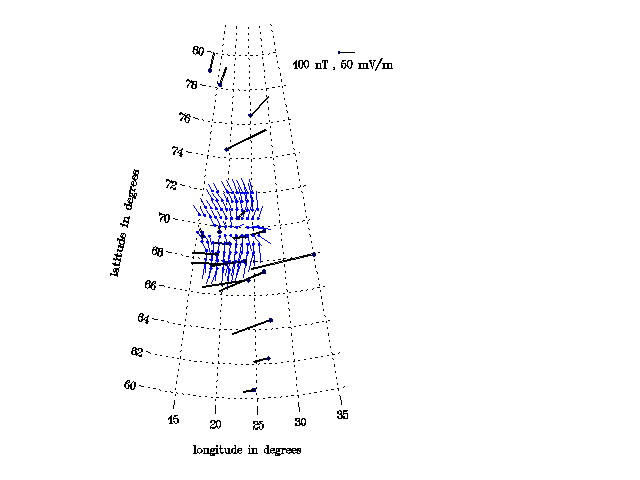Hall conductance distribution obtained by the method of characteristics: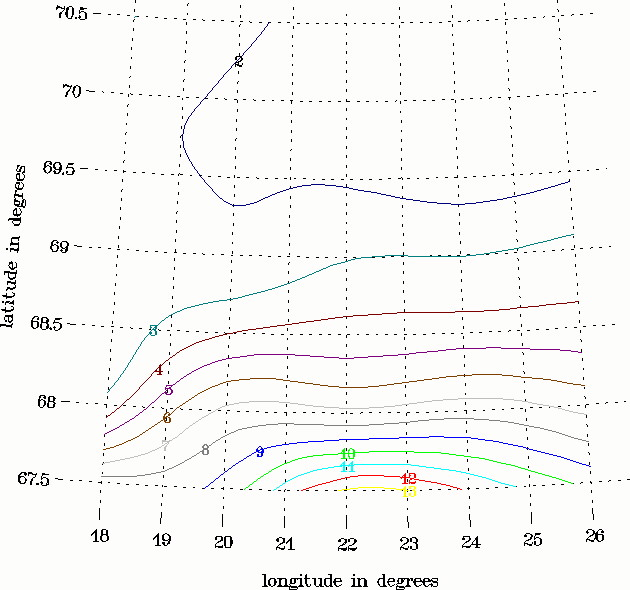Actual (not equivalent) horizontal ionospheric currents: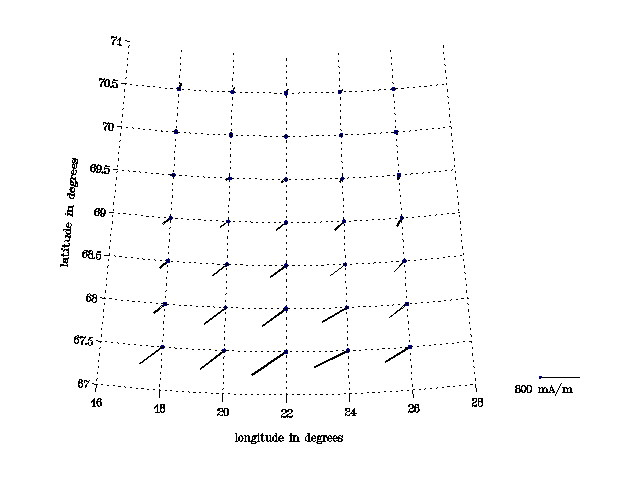Total field-aligned currents (FAC):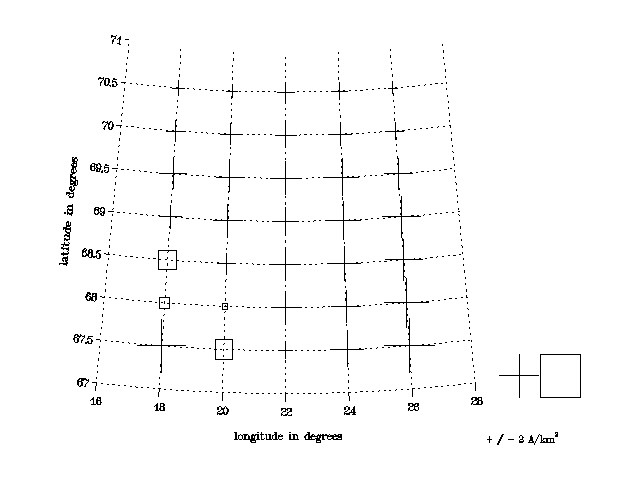With the results, much of detailed analysis can be done, as suitable for the respective study. Among others, Hall and Pedersen currents, FACs associated with them, curl-free and divergence-free parts of current systems, etc. can be calculated. The example below shows the FACs due to Sigma_P * div E for the situation as above:And here are the FACs due to (grad Sigma_P) * E: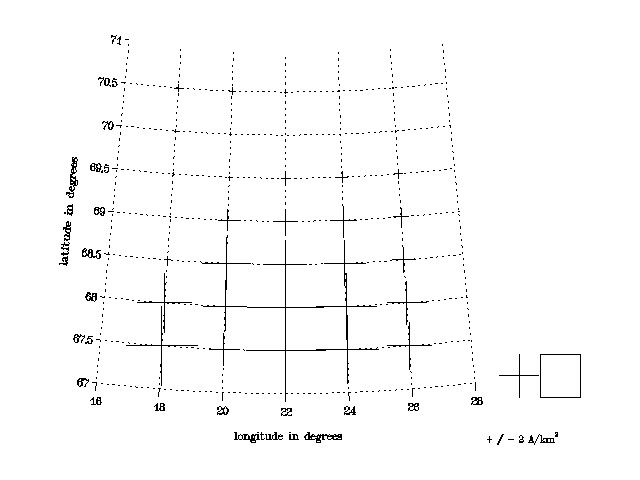For further questions and proposals of events to be analysed, contact Olaf Amm.

Maintained by: Olaf Amm
Latest update: 10. 5. 1999